# Introductory Algebra GlossaryPage 2

#### WATCH ALL SLIDES

Pairs of numbers whose product is 1:

1/3 and 3 are reciprocals.

Slide 15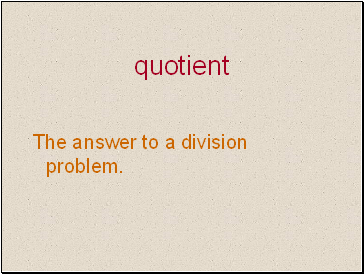## Quotient

The answer to a division problem.

Slide 16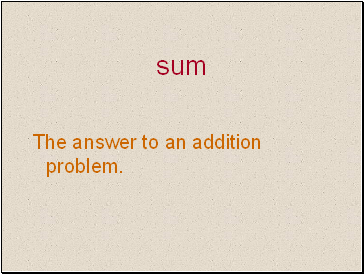## Sum

Slide 17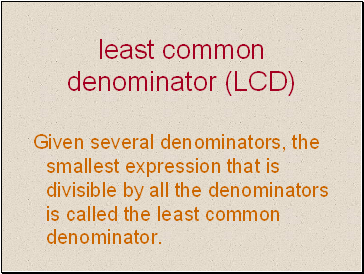## Least common denominator (LCD)

Given several denominators, the smallest expression that is divisible by all the denominators is called the least common denominator.

Slide 18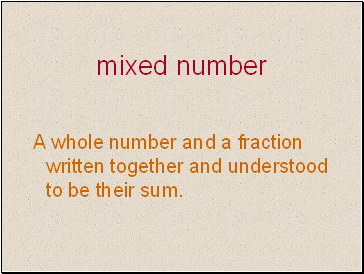## Mixed number

A whole number and a fraction written together and understood to be their sum.

Slide 19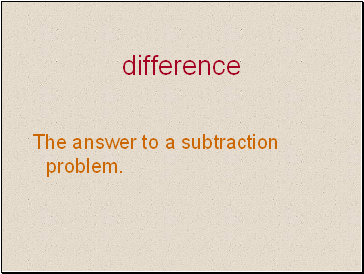## Difference

The answer to a subtraction problem.

Slide 20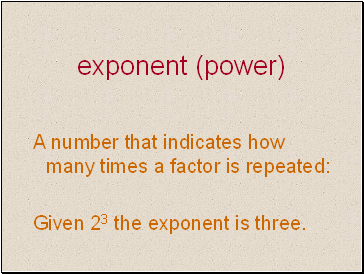## Exponent (power)

A number that indicates how many times a factor is repeated:

Given 23 the exponent is three.

Slide 21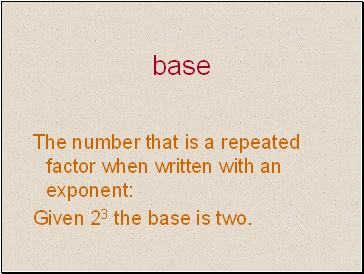## Base

The number that is a repeated factor when written with an exponent:

Given 23 the base is two.

Slide 22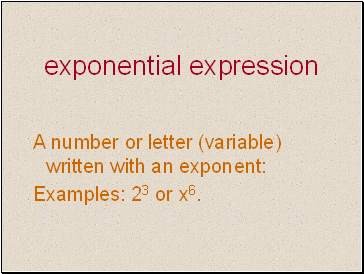## Exponential expression

A number or letter (variable) written with an exponent:

Examples: 23 or x6.

Slide 23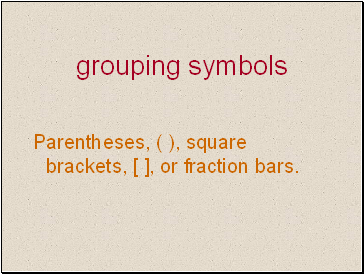## Grouping symbols

Parentheses, ( ), square brackets, [ ], or fraction bars.

Slide 24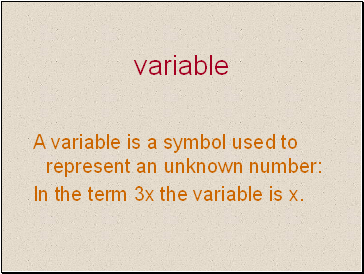## Variable

A variable is a symbol used to represent an unknown number:

In the term 3x the variable is x.

Slide 25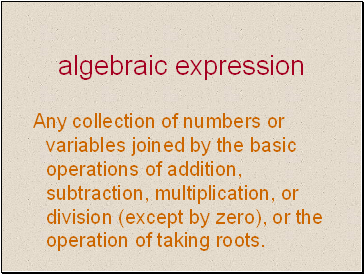## Algebraic expression

Any collection of numbers or variables joined by the basic operations of addition, subtraction, multiplication, or division (except by zero), or the operation of taking roots.

Slide 26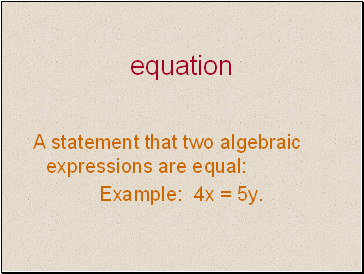## Equation

A statement that two algebraic expressions are equal:

Example: 4x = 5y.

Slide 27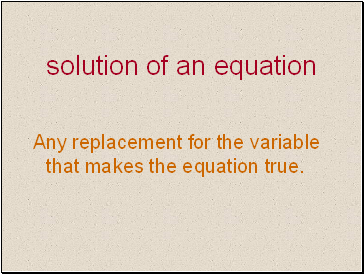## Solution of an equation

Any replacement for the variable that makes the equation true.

Slide 28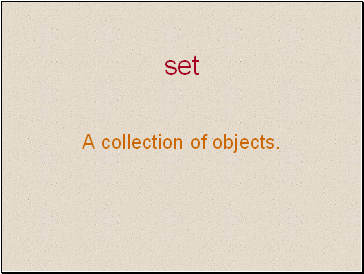## Set

A collection of objects.

Slide 29

Go to page:
1  2  3  4  5